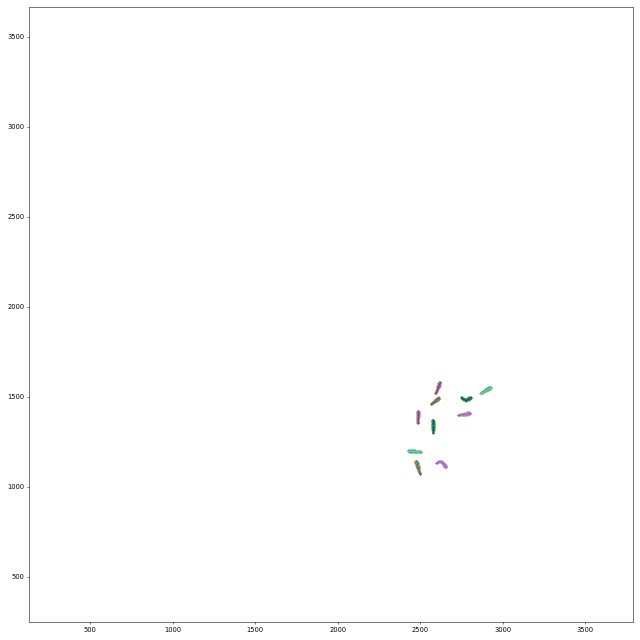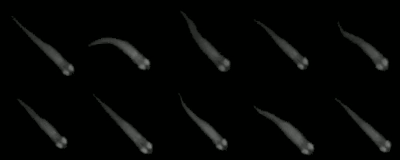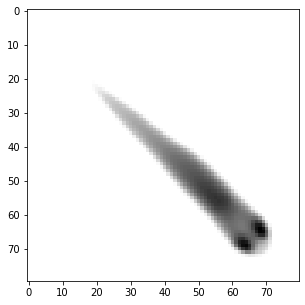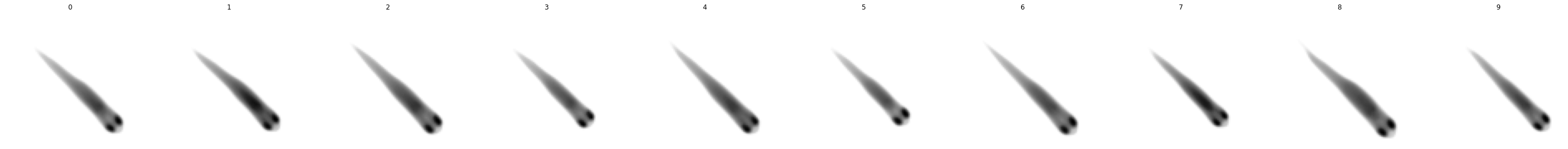# File formats

## Positional Data

Upon hitting the `S` key/clicking the export tracking data button in the menu, or when using the `auto_quit` option, TRex will save one file per individual. They are, by default, saved to `data/[VIDEONAME]_fish[NUMBER].npz` and contain all of the data fields selected from the export options.

Note

Variable names, user-specified or exported from TRex/TGrabs, can have attachments indicated by `#` (such as `X#wcentroid`). The text after `#` is the “source” for the data, of which there are four different ones:

• `#head` or empty (default): head position (only available if postures are calculated)

• `#wcentroid`: body center (based on weighted image/pixel information)

• `#centroid`: body center (center of mass of all thresholded pixels)

• `#pcentroid`: posture centroid (the center of the midline)

The resulting data can differ between data sources to varying degrees, depending on the posture detection, size and movement speed of individuals. This is most notable during sharp turns, where the body center (centroid) will remain fairly stable while the head position moves quickly (and thus also has higher SPEED).

Make sure you are using the most suitable one for your purposes.

Variable

Description

X,Y

Position on the X/Y-axis. Y starts on the top-left. Both are in cm (if `cm_per_pixel()` is set correctly, otherwise the conversion is based on an assumed width of 30cm for the arena). If `output_interpolate_positions()` is set, then this value can be an interpolated value (if missing is also 1).

missing

Set to 0 if the individual has data in this frame, or 1 otherwise.

SPEED

Magnitude of the first derivative of position (in cm/s).

VX,VY

X/Y-component of the first derivative of position (in cm/s).

AX,AY

X/Y-component of the acceleration in cm/s (if `cm_per_pixel()` is set correctly, otherwise the conversion is based on an assumed width of 30cm for the arena).

time

Time in seconds (floating point) from the start of the video, consistent across individuals.

timestamp

The time as a UNIX timestamp.

frame

Montonically increasing integer number, consistent across individuals. Note: when exporting to CSV/NPZ, the individual files do not have to start at frame `0`, and do not have to contain all frames from `0` to `N`.

num_pixels

The number of pixels in the blob (after thresholding).

ANGLE

The absolute angle in radians with respect to the X-axis of the image (e.g. 0rad indicates the individual is horizontal, looking to the right). You can convert this to degrees using ANGLE/pi*180.

blobid

Each objects gets a (more or less) unique identifier per frame, based on its position and size. This ID is saved here, and is what’s used for manual assignments of individuals to objects, for example.

midline_length

The length of the midline for the given frame (in pixels) - the sum of all euclidean distances between midline segments.

outline_size

The number of points in the outline of the individual. This can be used as a coarse approximation of individual size.

consecutive_segment_id

Each consecutively tracked frame segments gets an ID, which is returned here.

average_category

This applies only to videos where visual categorization of individuals has been applied. Here, the average category represents the predicted class for this individual, within the current consecutively tracked frame segment.

category

As above, but not averaged and instead saved individually per frame.

BORDER_DISTANCE

Usually this is the distance from the centroid of the individual to the edge of the image - in case a `use_circle_mask()` has been used during conversion, the circle is used as an approximation of the tank size.

NEIGHBOR_DISTANCE

Average euclidean distance to neighbors (in cm).

MIDLINE_OFFSET

The offset of the tail of an individual to the center line through its body (roughly speaking). More specifically, it is the y-coordinate of the tail tip, after normalizing the orientation and position of the individual (as in the posture window).

Each of these metrics is saved per frame, meaning that each metric mentioned here has the same number of values per individual. However, not all individuals have the same number of frames and do not necessarily start at the same time (even though the time variable is consistent across individuals). If an individual is first detected later in the video (not in the first frame), then that frame is the first one to appear in the exported data. Individuals may also not appear towards the end of the video, in which case there also won’t be any data exported for that part.

Note

There are a couple hashtags in there – these simply mean that the data-source for that metric is different. For example, `wcentroid` means that this metric is centered on the centroid (weighted by pixel values) of each individual. If no hashtag is provided, the metric centers on the head of each individual. These are usually closely related, but are different from each other e.g. when the individual moves its head independently from other parts of the body. There the head-based metric would show much more wiggling around than the centroid-based metric.

If one metric (such as anything posture-related) is not available in a frame – either because the individual was not found, or because no valid posture was found – then all affected metrics (sometimes all) will be set to `infinity`. A typical way of opening such a file and plotting a trajectory would be:

```import numpy as np
import matplotlib.pyplot as plt

# sample output: ['threshold_reached', 'num_pixels', 'time',     \
#     'midline_length', 'frame', 'Y#wcentroid', 'Y', 'missing',  \
#     'X', 'SPEED', 'SPEED#pcentroid', 'MIDLINE_OFFSET',         \
#     'X#wcentroid', 'SPEED#wcentroid']
print(npz.files)

X = npz["X#wcentroid"]
Y = npz["Y#wcentroid"]

# sample output: (30269,)
# just a stream of X positions for fish0
print(X.shape)

# using the mask gets rid of np.inf values (otherwise the plot
# might get weird). "missing" is 1 whenever the individual is
# not tracked in that frame:

# plot a connected trajectory for fish0:
plt.figure(figsize=(5,5))
plt.show()
```

## Posture-data

For each individual (as long as `output_posture_data()` is set to `true`), TRex writes a file `[output_path]/[FILENAME]_posture_*.npz` containing the following fields:

Name

Description

frames

Frame index for all data contained in all other arrays.

offset

Top-left corner (px) of the object that this posture was generated from.

midline_lengths

Number of points in the midline.

midline_centimeters

Length of the midline in cms (based on the `cm_per_pixel()` parameter)

midline_offsets

A bit obscure: Angle (rad) from start to end of the midline (line through first and last point).

midline_angle

Angle (rad) of a line from head-position (first midline segment) through the midline segment at a fraction of `midline_stiff_percentage()` of the midline - approximating the heading of the individual.

posture_area

Area of the polygon spanned by the outline points (calculated using the Shoe lace formula).

midline_points

2D Points (px) of the midline in real-world coordinates.

midline_points_raw

2D Points (px) of the midline in normalized coordinates.

outline_lengths

Number of points in the outline.

outline_points

Each outline point consists of X and Y, but each outline can be of a different length. To iterate through these points, one must keep a current index that increases by `outline_lengths[frame]` per frame.

For an example of how to use this data, have a look at this example from the repository. It demonstrates loading all posture files from one video, drawing the posture output in matplotlib and saving it to a new movie file. This is what the output could look like:## Tracklet images

Tracklet images can be quite useful when a different software has to perform operations on individual images (or summary-images per segment), like, for example, using DeepLabCut or DeepPoseKit to estimate poses in more detail – after tracking and visual identification in TRex have ensured that we know who is whom. Interoperability between the tools can have other advantages, too. Images can be normalized, for example, and become easier to annotate manually – these altered video sequences can be saved for each individual.The container `<VIDEO>_tracklet_images.npz` contains summaries of all consecutive segments for each individual in the form of `['images', 'meta']`, where `meta` is matrix of `Nx3` (where `N` is the number of segments). The three columns are ID, segment start and segment end (frame numbers). Images is a matrix of `NxWxH` depending on the image dimensions set in `recognition_image_size()`.

```import numpy as np
import matplotlib.pyplot as plt
```

### Open all images of a certain individual ID

```with np.load("Videos/data/video_tracklet_images_single_part0.npz") as npz:
print(npz.files)

images = npz["images"]  # load images
ids    = npz["ids"]     # ids have the same length as images
frames = npz["frames"]  # the frame for each row

# so we can use them as a mask for the images array:
print(images[ids == 0].shape)

# now draw a median image of this fish. since it is normalized (orientation),
# it will be a nice, clean picture of what it looks like most of the time.
# if it does not, then your posture settings are probably off.
# this only works after successful visual identification + correction of course.
plt.figure(figsize=(5,5))
plt.imshow(np.median(images[ids == 0], axis=0), cmap="Greys")
plt.show()
```
```['images', 'frames', 'ids']
(19247, 80, 80)
```### Now we want to see that for all individuals

But we are using the meta tracklet pack for this. It contains only one image per consecutive segment.

```with np.load("Videos/data/video_tracklet_images.npz") as npz:
meta = npz["meta"]
N = len(np.unique(meta[:, 0])) # how many fish do we have here?

# plot all individuals in a row. this will probably be real tiny for many more individuals.
f, axes = plt.subplots(1, N, figsize=(5*N, 5))
for ax, i in zip(axes, np.unique(meta[:, 0])):
ax.axis('off')
ax.imshow(np.median(npz["images"][meta[:, 0] == i], axis=0), cmap="Greys")
ax.set_title(str(i))
plt.show()
```### We can now map from segments (meta) to tracklet images from the big file

```for ID, start, end in meta:
mask = np.logical_and(ids == ID, np.logical_and(frames >= start, frames <= end))
```
```0 0 - 40 (41, 80, 80)
0 42 - 50 (9, 80, 80)

[...]

9 19235 - 19242 (8, 80, 80)
9 19245 - 19251 (7, 80, 80)
9 19252 - 19305 (54, 80, 80)
9 19306 - 19316 (11, 80, 80)
```

## Visual fields

Visual fields are saved by clicking the respective menu button (“export visual fields”) in TRex. This will save one or multiple files, depending on file size. If the files are bigger than ~3GB, then they have to be saved separately in individual .npy files - otherwise they will all be merged into one .npz container called e.g. `data/<VIDEONAME>_visual_field_fish0.npz`.

Each container holds multiple arrays, each are shaped `Nx2x2x512` for `N` frames, 2 eyes and 2 depth-layers per eye:

• depth: distance to the visible individual (or `INFINITY == none`; see also this github issue)

• ids: id of the visible individual (or `-1` for self-intersection)

• body_part: a percentage that indicates how far from the head (and how close to the tail) the point on the seen individual is on its outline

as well as some meta data:

• colors: (`Mx4`): ID + RGB for M individuals

• fov_range (`2x1`): FOV range in radians

• frame_range (`2x1`): Start and end of exported region

• fish_pos (`Nx2`): XY position of the individual for each frame

• fish_angle (`Nx1`): angle of the body relative to the x-axis

• eye_pos (`Nx2x2`): XY position for each eye

• eye_angle (`Nx2`): angle for each eye

• frames (`Nx1`): frame index

(Todo)

## PreprocessedVideo (pv)

Videos in the PV format are structured as follows:

```**[HEADER SECTION]**
(string) "PV" + (version_nr)
(byte)   channels
(uint16) width
(uint16) height
(Rect2i) four ushorts with the mask-offsets left,top,right,bottom
(uchar)  sizeof(HorizontalLine)
(uint32) number of frames
(uint64) pointer to index at the end of file
(uint64) timestamp (time since 1970 in microseconds)
(string) project name
(byte*)  average img ([width x height] x channels)

**[DATA SECTION]**
for each frame:
(uchar) compression flag (if 1, the whole frame is compressed)
if compressed:
(uint32) original size
(uint32) compressed size
(byte*) lzo1x compressed data (see below for uncompressed)
else:
[UNCOMPRESSED DATA PER FRAME] {
(uint64) timestamp (in microseconds) since start of movie
(uint16) number of individual cropped images

for each object/blob:
(uint16) y of first HorizontalLine
(uint16) (n)umber of HorizontalLine structs
(byte*)  n * sizeof(HorizontalLine)
(byte*)  original image pixels ordered exactly as in HorizontalLines (BGR, CV_8UC(n))
}

**[INDEX TABLE]**
for each frame
(uint64) frame start position in file

```(uint16) x0